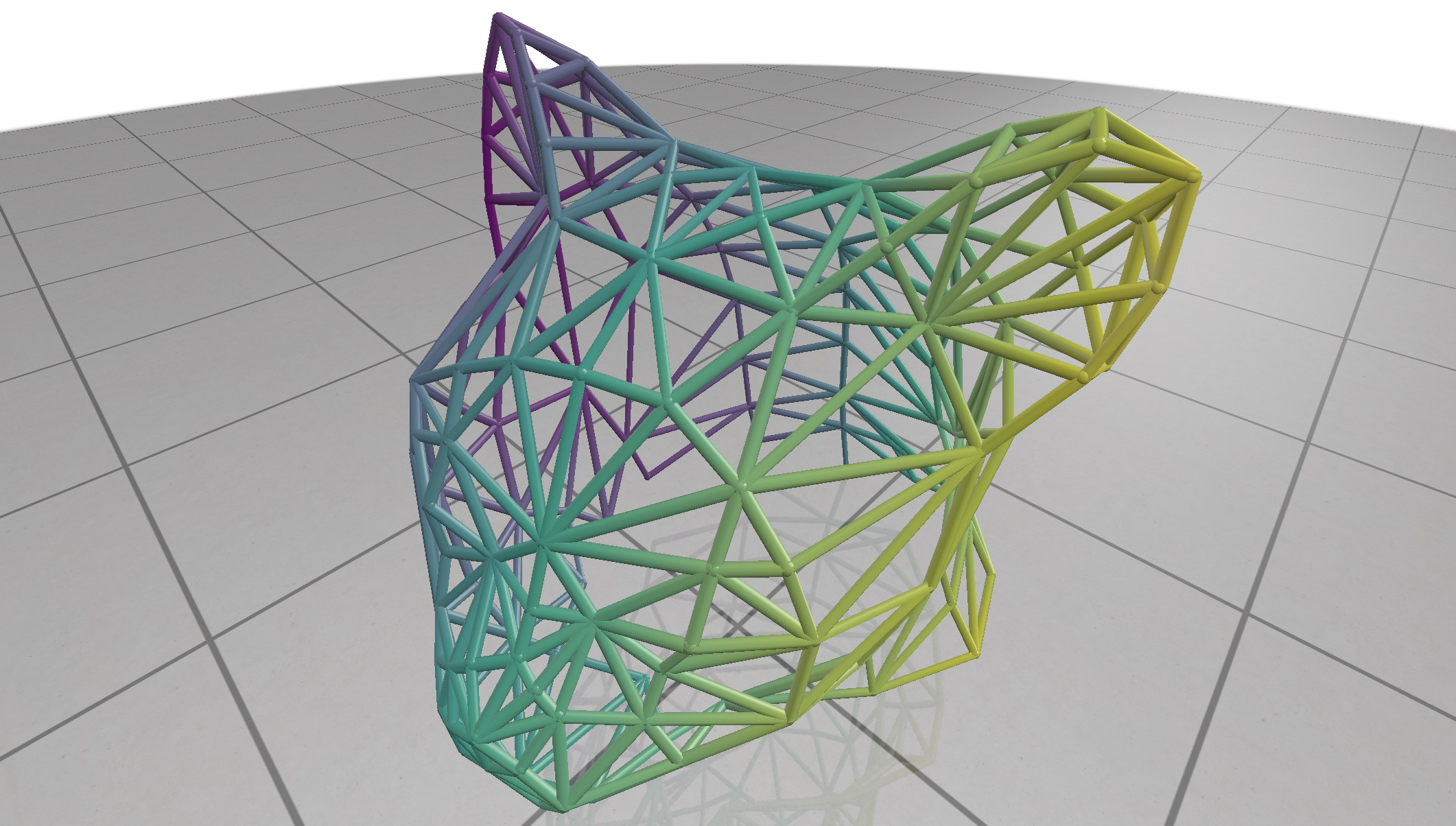# Scalar Quantities

Visualize scalar valued data at the nodes or edges of a curve network.Example:

#include "polyscope/curve_network.h"

std::vector<double> xC(nodes.size());
for (size_t i = 0; i < nodes.size(); i++) {
xC[i] = nodes[i].x; // (use the x coordinate as sample data)
}

// visualize

CurveNetwork::addNodeScalarQuantity(std::string name, const T& values, DataType type = DataType::STANDARD)
• values is the array of scalars at nodes. The type should be adaptable to a float scalar array. The length should be the number of nodes in the curve network.
CurveNetwork::addEdgeScalarQuantity(std::string name, const T& values, DataType type = DataType::STANDARD)
• values is the array of scalars at edges . The type should be adaptable to a float scalar array. The length should be the number of edges in the curve network.